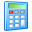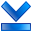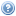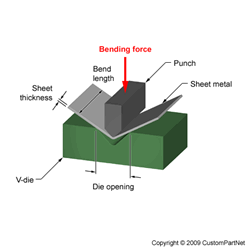Widgets# V-Bending Force Calculator

Calculate the required force to bend a piece of sheet metal using V-bending, a process in which a V-shaped punch presses the sheet into a V-die. The bending force can be calculated from the sheet thickness, die opening, bend length, and the ultimate tensile strength of the material. The die ratio may be entered to calculate the die opening, which is typically 6 to 18 times the sheet thickness. The calculated tonnage can be used for the selection of a machine, such as a press brake, to perform the bending operation. Learn more about Bending.

## V-Bending Force Calculator

Sheet thickness (in):